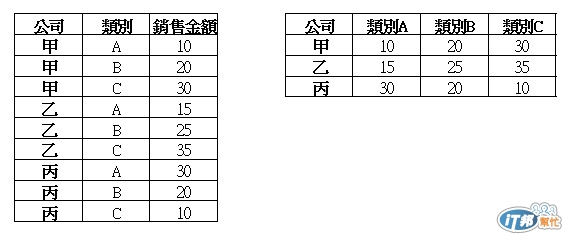#0

## T-SQL SELECT 欄列顯示排列問題

SELECT顯示如圖右

``````SELECT 公司 ,
ISNULL(CAST(SUM(CASE WHEN 類別 LIKE 'A%' THEN 銷售金額 END) AS VARCHAR),'') AS 'A類' ,
ISNULL(CAST(SUM(CASE WHEN 類別 LIKE 'B%' THEN 銷售金額 END) AS VARCHAR),'') AS 'B類' ,
ISNULL(CAST(SUM(CASE WHEN 類別 LIKE 'C%' THEN 銷售金額 END) AS VARCHAR),'') AS 'C類'
FROM 銷售檔
``````kradark iT邦好手 1 級 ‧ 2012-03-29 23:43:23 檢舉

### 1 個回答

6

``````&lt;pre class="c" name="code">
SELECT [公司],('類別' + [類別]) AS [類別],[銷售金額] INTO #tmp FROM [tb1]
GO
DECLARE @SQL NVARCHAR(MAX)
COALESCE(
@ColHeader + ',[' +  CAST([類別] AS varchar) + ']',
'[' + CAST([類別] AS varchar) + ']'
)
FROM (SELECT DISTINCT [類別] FROM #tmp) AS x
SET @SQL = N'
SELECT * FROM #tmp PIVOT (SUM([銷售金額]) FOR [類別] IN (' + @ColHeader + ')) AS p'
EXECUTE(@SQL)
DROP TABLE #tmp
``````